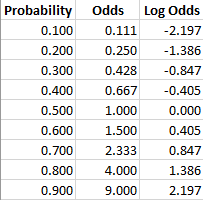# Log Odds: Simple Definition & Examples, Conversions

Log odds play a central role in logistic regression. Every probability can be easily converted to log odds, by finding the odds ratio and taking the logarithm.

Despite the relatively simple conversion, log odds can be a little esoteric. Jaccard (2001, p.10) calls them “…counterintuitive and challenging to interpret,” especially if you don’t have a strong statistical background. That said, the formulas are relatively simple, even if the results are a little challenging to decipher.

## Conversions: Probability to Odds to Log of Odds

Probability, odds ratios and log odds are all the same thing, just expressed in different ways. It’s similar to the idea of scientific notation: the number 1,000 can be written as 1.0*103 or even 1*10*10*10. What works for one person, or one equation, might not work for another. In many cases, you can simply choose which format you want to use. Other times (for example, you’re publishing a paper or are using logistic regression), you might be forced to adopt a particular format.

Probability is the probability an event happens. For example, there might be an 80% chance of rain today.
Odds (more technically the odds of success) is defined as probability of success/probability of failure. So the odds of a success (80% chance of rain) has an accompanying odds of failure (20% chance it doesn’t rain); as an equation (the “odds ratio“), that’s .8/.2 = 4.
Log odds is the logarithm of the odds. Ln(4) = 1.38629436 ≅ 1.386.

Conversion to log odds results in symmetry around zero, which is easier for analysis, as shown in the following table (Jaccard, 2001):## Log Odds and the Logit Function

The odds ratio is the probability of success/probability of failure. As an equation, that’s P(A)/P(-A), where P(A) is the probability of A, and P(-A) the probability of ‘not A’ (i.e. the complement of A).

Taking the logarithm of the odds ratio gives us the log odds of A, which can be written as

log(A) = log(P(A)/P(-A)),

Since the probability of an event happening, P(-A) is equal to the probability of an event not happening, 1 – P(A), we can write the log odds as

log [p/(1-p)]

Where:

• p = the probability of an event happening
• 1 – p = the probability of an event not happening

When a function’s variable represents a probability, p (as in the function above), it’s called the logit function .

## Using Log Odds

We sometimes choose to use log odds instead of more basic probability measures because they’re so easily updated with new data.

For instance, suppose you have a 5% chance that a thief will come in at your door on any given night. You have a watch dog, but he’s not terribly reliable; he’ll bark half the time if a thief comes, and just 1/4 of the time if the person walking by is an honest man. Now imagine you hear footsteps, and the dog barks.

Before your dog barked, the log odds of a thief were ln(.05/.95) = ln(1/19), or -2.9444. We call that your prior log odds for the thief. The likelihood ratio of a bark is just the probability of a bark with a thief (1/2) over the likelihood of a bark with no thief (1/4), and to find the log odds we take the log of that: ln((1/2)/(1/4)) = log(2) = 0.6931. Now the posterior log odds of the thief—the log odds that there is a thief, given you’ve just heard the dog bark—is -2.9444 + 0.6931, or -2.2513.

Since the ln (odds ratio) = log odds, elog odds = odds ratio. So to turn our -2.2513 above into an odds ratio, we calculate e-2.2513, which happens to be about 0.1053:1. So the probability we have a thief is 0.1053/1.1053 = 0.095, so 9.5 %. Notice how we converted the odds ratio to a probability by dividing the first part of the ratio with the sum of both parts (the total). Notice also that we came to our final answer without any involved calculations, assuming, of course, we have a calculator to help us with the logarithms.

We’ve used natural logs here (base e); you can actually use logs in any base, you just need to be consistent.

## References

Eckel, S. (2008). Interpreting Logistic Regression Models. http://www-hsc.usc.edu/~eckel/biostat2/notes/notes14.pdf
Jaccard, J. (2001) Interaction Effects in Logistic Regression, Issue 135. SAGE.
Rotella, J. (n.d.) Probability, Log Odds, and Odds. Retrieved December 14, 2017 from: http://www.montana.edu/rotella/documents/502/Prob_odds_log-odds.pdf

CITE THIS AS:
Stephanie Glen. "Log Odds: Simple Definition & Examples, Conversions" From StatisticsHowTo.com: Elementary Statistics for the rest of us! https://www.statisticshowto.com/log-odds/
---------------------------------------------------------------------------Need help with a homework or test question? With Chegg Study, you can get step-by-step solutions to your questions from an expert in the field. Your first 30 minutes with a Chegg tutor is free!